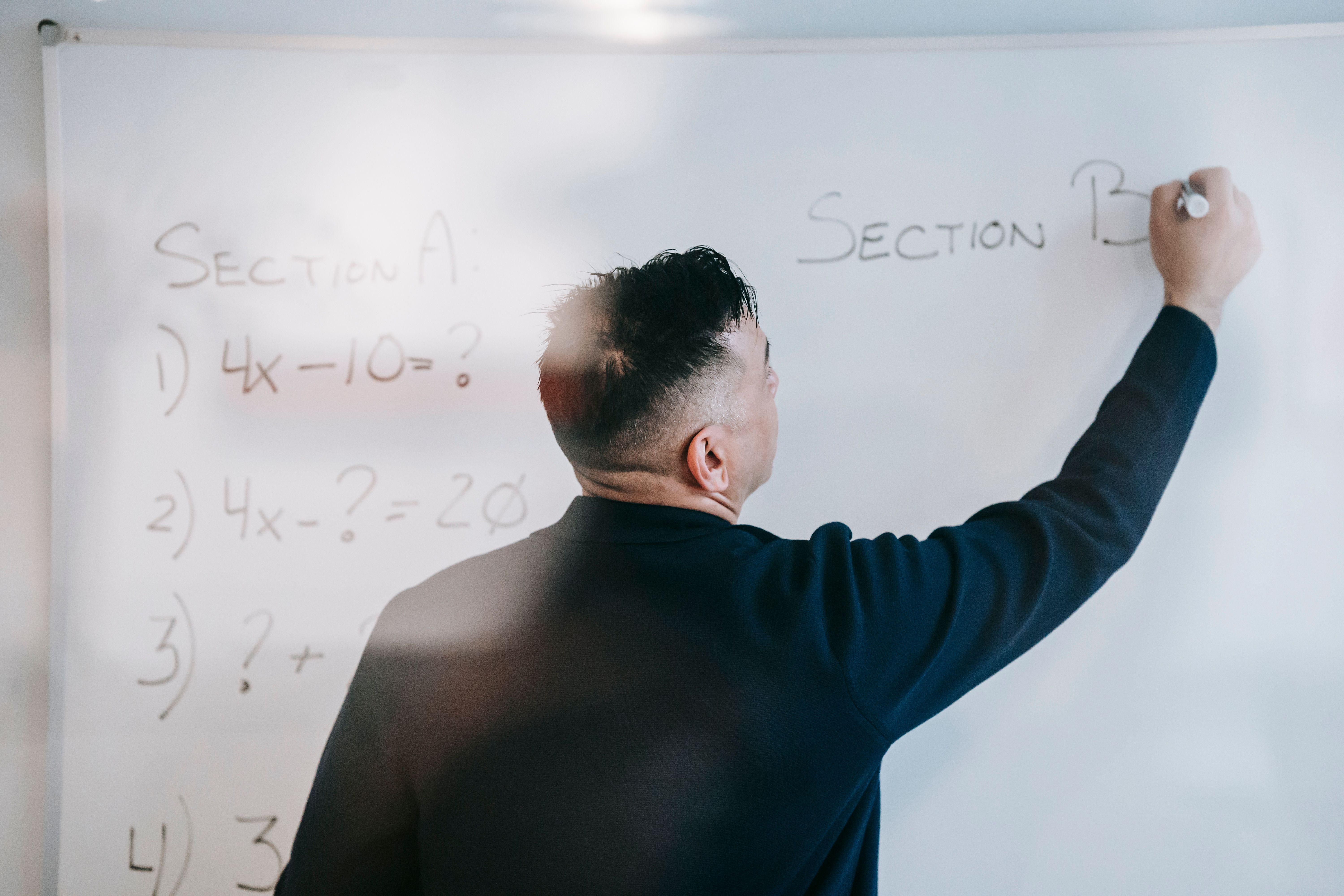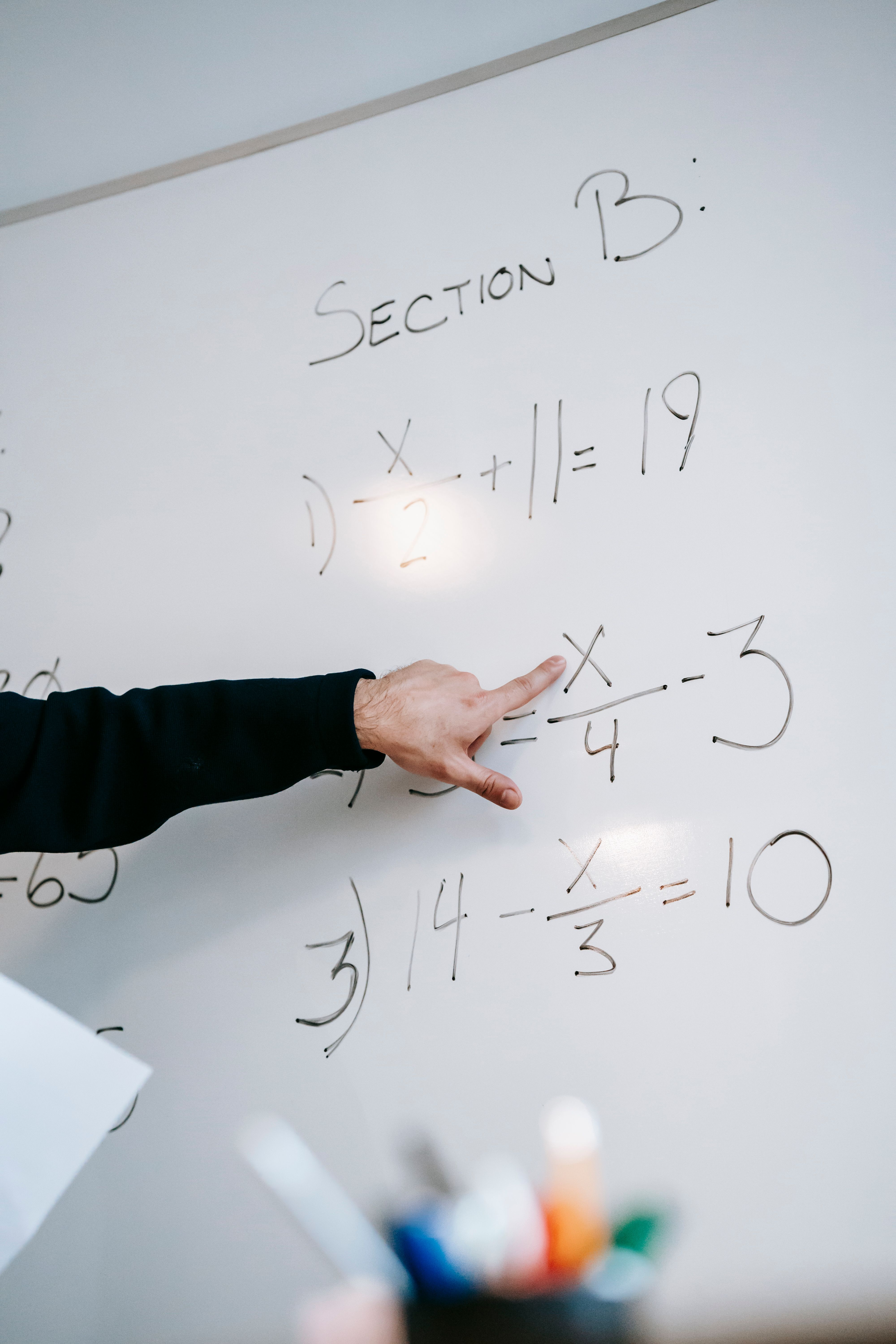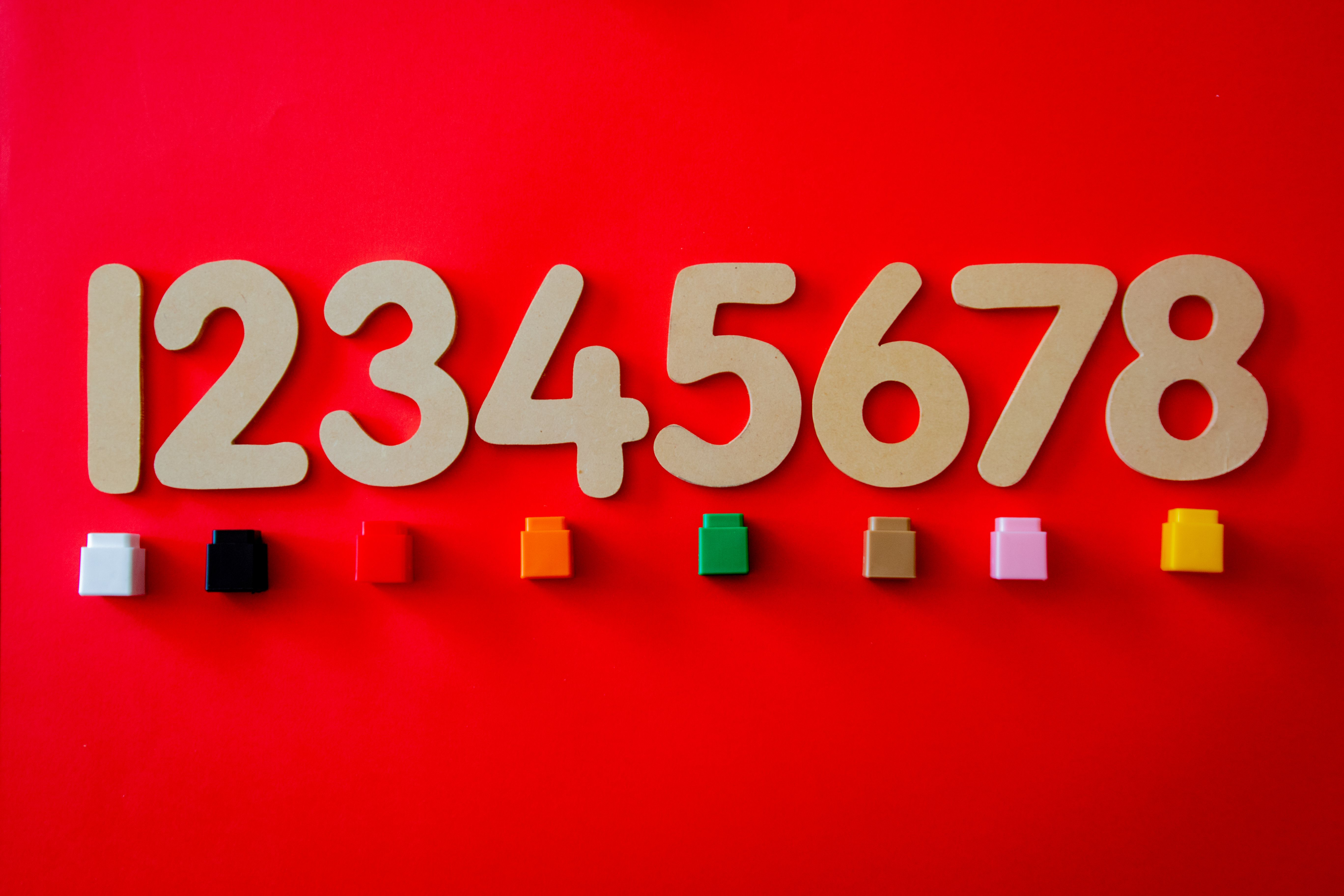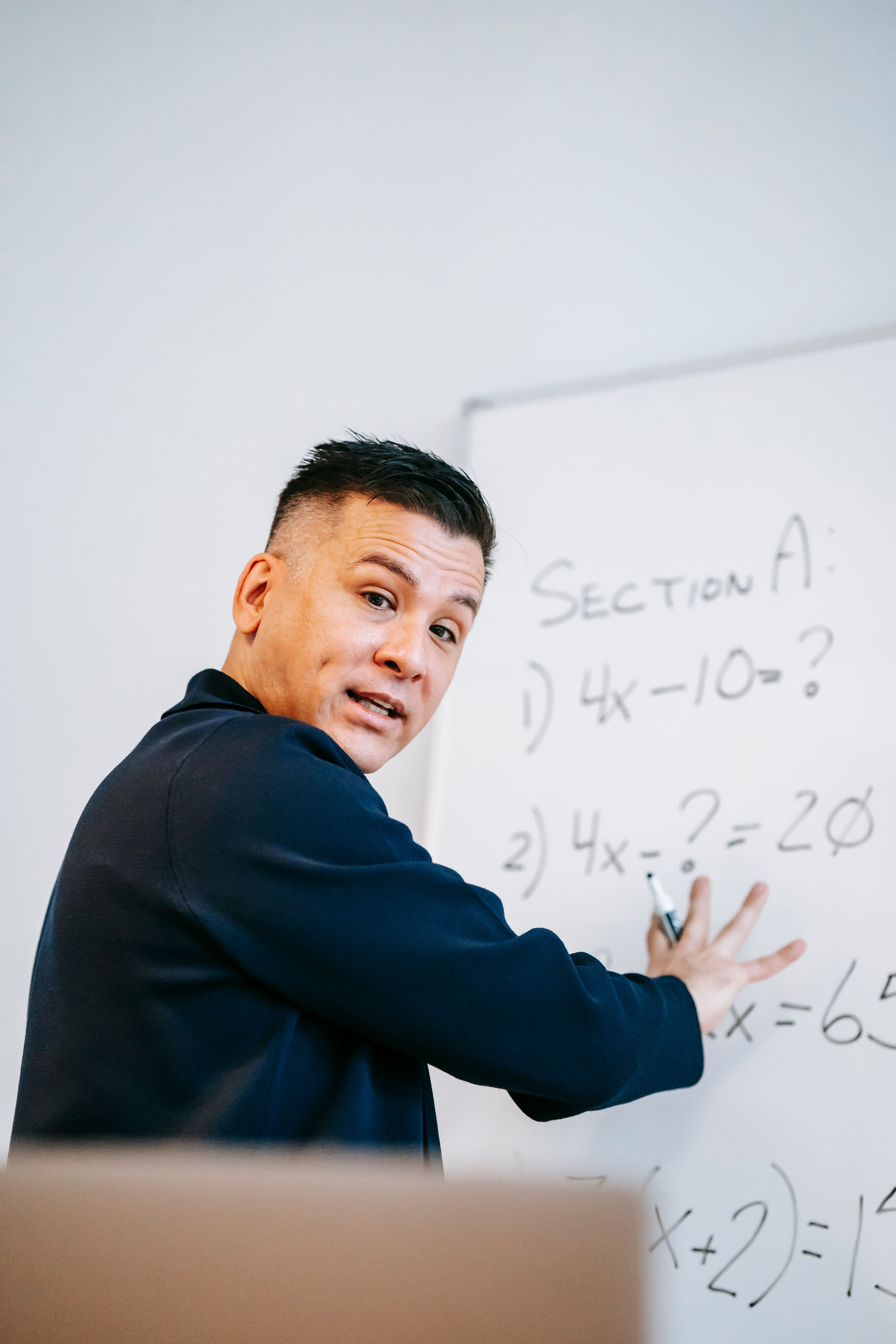# Is discrete math supposed to be hard?

Is Discrete Math Supposed to be Hard?

# Why is Discrete Math Considered a Difficult Subject?Discrete math is a subject that is required for many college majors, particularly in computer science and mathematics. However, despite its importance, it is often considered a difficult subject by students. In this article, we will explore the reasons why discrete math is challenging and provide tips on how to approach it.

## FAQ

### What is discrete math?

Discrete math is the study of mathematical structures that are countable and separable. It includes topics such as set theory, graph theory, combinatorics, and logic.

### Why is discrete math difficult?

There are several reasons why discrete math can be challenging:

#### Abstract Nature

Discrete math deals with abstract concepts that are not always easy to visualize or relate to the physical world. For example, in set theory, we work with sets of objects that are not necessarily physical, such as the set of all even numbers. This can make it difficult for some students to understand the relevance of the concepts and how they apply.

#### Unfamiliar Notation

Discrete math often uses mathematical notation that is different from what students are used to seeing in high school math classes. For example, symbols such as ∀ and ∃ are used to represent quantifiers, and set notation is used extensively. It can take time to become familiar with these notations and understand how they are used.

#### Multiple Problem-Solving Strategies

Discrete math problems often require multiple problem-solving strategies to be applied in order to arrive at a solution. This can include techniques such as proof by contradiction, mathematical induction, and combinatorial reasoning. Being able to choose the right strategy for a specific problem and apply it correctly can be challenging.### How can I approach discrete math?

Here are some tips for approaching discrete math:

#### Practice, Practice, Practice

Discrete math, like any other subject, requires practice to master. Completing practice problems regularly will help solidify your understanding of the concepts and improve your problem-solving skills. Many textbooks and online resources offer practice problems and solutions, so take advantage of them.

#### Understand the Concepts, Not Just the Formulas

It is important to understand the concepts behind the formulas and problem-solving techniques in discrete math, rather than just memorizing them. This will help you apply the concepts to a variety of problems and think more critically about the material.

#### Ask for Help When You Need It

If you are struggling with discrete math, don’t be afraid to ask for help. Many universities offer tutoring services or have math departments that can provide additional support. Additionally, there are online communities and forums where you can ask questions and get help from peers and experts.